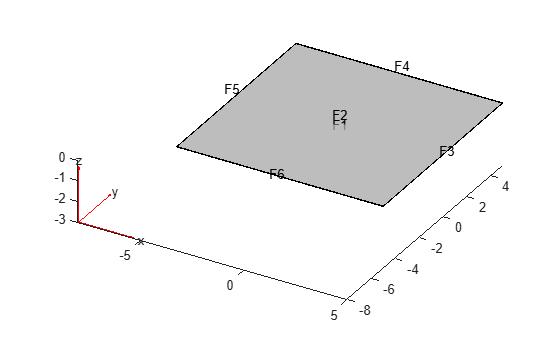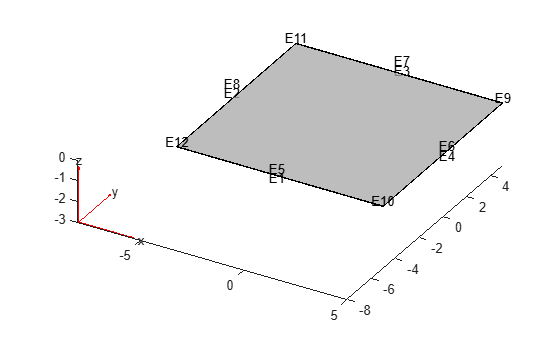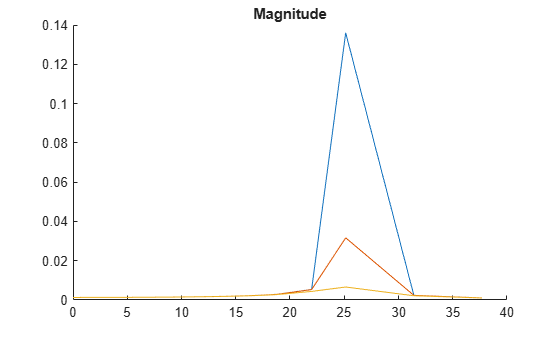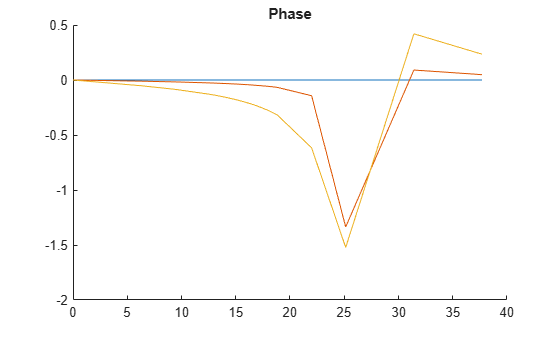FrequencyStructuralResults

Frequency response structural solution and its derived quantities

Description

A FrequencyStructuralResults object contains the displacement, velocity, and acceleration in a form convenient for plotting and postprocessing.

Displacement, velocity, and acceleration are reported for the nodes of the triangular or tetrahedral mesh generated by generateMesh. The displacement, velocity, and acceleration values at the nodes appear as FEStruct objects in the Displacement, Velocity, and Acceleration properties. The properties of these objects contain the components of the displacement, velocity, and acceleration at the nodal locations.

To evaluate the stress, strain, von Mises stress, principal stress, and principal strain at the nodal locations, use evaluateStress, evaluateStrain, evaluateVonMisesStress, evaluatePrincipalStress, and evaluatePrincipalStrain, respectively.

To evaluate the reaction forces on a specified boundary, use evaluateReaction.

To interpolate the displacement, velocity, acceleration, stress, strain, and von Mises stress to a custom grid, such as the one specified by meshgrid, use interpolateDisplacement, interpolateVelocity, interpolateAcceleration, interpolateStress, interpolateStrain, and interpolateVonMisesStress, respectively.

For a frequency response model with damping, the results are complex. Use functions such as abs and angle to obtain real-valued results, such as the magnitude and phase. See Solution to Frequency Response Structural Model with Damping.

Creation

Solve a frequency response problem by using the solve function. This function returns a frequency response structural solution as a FrequencyStructuralResults object.

Properties

expand all

Properties of an FEStruct object are read-only.

Displacement values at the nodes, returned as an FEStruct object. The properties of this object contain the components of the displacement at the nodal locations.

Velocity values at the nodes, returned as an FEStruct object. The properties of this object contain the components of the velocity at the nodal locations.

Acceleration values at the nodes, returned as an FEStruct object. The properties of this object contain the components of the acceleration at the nodal locations.

Solution frequencies, returned as a real vector. SolutionFrequencies is the same as the flist input to solve.

Data Types: double

Finite element mesh, returned as a FEMesh object. For details, see FEMesh Properties.

Object Functions

 evaluateStress Evaluate stress for dynamic structural analysis problem evaluateStrain Evaluate strain for dynamic structural analysis problem evaluateVonMisesStress Evaluate von Mises stress for dynamic structural analysis problem evaluateReaction Evaluate reaction forces on boundary evaluatePrincipalStress Evaluate principal stress at nodal locations evaluatePrincipalStrain Evaluate principal strain at nodal locations interpolateDisplacement Interpolate displacement at arbitrary spatial locations interpolateVelocity Interpolate velocity at arbitrary spatial locations for all time or frequency steps for dynamic structural model interpolateAcceleration Interpolate acceleration at arbitrary spatial locations for all time or frequency steps for dynamic structural model interpolateStress Interpolate stress at arbitrary spatial locations interpolateStrain Interpolate strain at arbitrary spatial locations interpolateVonMisesStress Interpolate von Mises stress at arbitrary spatial locations

Examples

collapse all

Solve a frequency response problem with damping. The resulting displacement values are complex. To obtain the magnitude and phase of displacement, use the abs and angle functions, respectively. To speed up computations, solve the model using the results of modal analysis.

Modal Analysis

Create a modal analysis model for a 3-D problem.

modelM = createpde('structural','modal-solid');

Create the geometry and include it in the model.

gm = multicuboid(10,10,0.025);
modelM.Geometry = gm;

Generate a mesh.

msh = generateMesh(modelM);

Specify the Young's modulus, Poisson's ratio, and mass density of the material.

structuralProperties(modelM,'YoungsModulus',2E11, ...
'PoissonsRatio',0.3, ...
'MassDensity',8000);

Identify faces for applying boundary constraints and loads by plotting the geometry with the face and edge labels.

pdegplot(gm,'FaceLabels','on','FaceAlpha',0.5)figure
pdegplot(gm,'EdgeLabels','on','FaceAlpha',0.5)Specify constraints on the sides of the plate (faces 3, 4, 5, and 6) to prevent rigid body motions.

structuralBC(modelM,'Face',[3,4,5,6],'Constraint','fixed');

Solve the problem for the frequency range from -Inf to 12*pi.

Rm = solve(modelM,'FrequencyRange',[-Inf,12*pi]);

Frequency Response Analysis

Create a frequency response analysis model for a 3-D problem.

modelFR = createpde('structural','frequency-solid');

Use the same geometry and mesh as you used for the modal analysis.

modelFR.Geometry = gm;
modelFR.Mesh = msh;

Specify the same values for the Young's modulus, Poisson's ratio, and mass density of the material.

structuralProperties(modelFR,'YoungsModulus',2E11, ...
'PoissonsRatio',0.3, ...
'MassDensity',8000);

Specify the same constraints on the sides of the plate to prevent rigid body modes.

structuralBC(modelFR,'Face',[3,4,5,6],'Constraint','fixed');

Specify the pressure loading on top of the plate (face 2) to model an ideal impulse excitation. In the frequency domain, this pressure pulse is uniformly distributed across all frequencies.

First, solve the model without damping.

flist = [0,1,1.5,linspace(2,3,100),3.5,4,5,6]*2*pi;
RfrModalU = solve(modelFR,flist,'ModalResults',Rm);

Now, solve the model with damping equal to 2% of critical damping for all modes.

structuralDamping(modelFR,'Zeta',0.02);
RfrModalAll = solve(modelFR,flist,'ModalResults',Rm);

Solve the same model with frequency-dependent damping. In this example, use the solution frequencies from flist and damping values between 1% and 10% of critical damping.

omega = flist;
zeta = linspace(0.01,0.1,length(omega));
zetaW = @(omegaMode) interp1(omega,zeta,omegaMode);
structuralDamping(modelFR,'Zeta',zetaW);

RfrModalFD = solve(modelFR,flist,'ModalResults',Rm);

Interpolate the displacement at the center of the top surface of the plate for all three cases.

iDispU = interpolateDisplacement(RfrModalU,[0;0;0.025]);
iDispAll = interpolateDisplacement(RfrModalAll,[0;0;0.025]);
iDispFD = interpolateDisplacement(RfrModalFD,[0;0;0.025]);

Plot the magnitude of the displacement. Zoom in on the frequencies around the first mode.

figure
hold on
plot(RfrModalU.SolutionFrequencies,abs(iDispU.Magnitude));
plot(RfrModalAll.SolutionFrequencies,abs(iDispAll.Magnitude));
plot(RfrModalFD.SolutionFrequencies,abs(iDispFD.Magnitude));
title('Magnitude')
xlim([10 25])
ylim([0 0.5])Plot the phase of the displacement.

figure
hold on
plot(RfrModalU.SolutionFrequencies,angle(iDispU.Magnitude));
plot(RfrModalAll.SolutionFrequencies,angle(iDispAll.Magnitude));
plot(RfrModalFD.SolutionFrequencies,angle(iDispFD.Magnitude));
title('Phase')